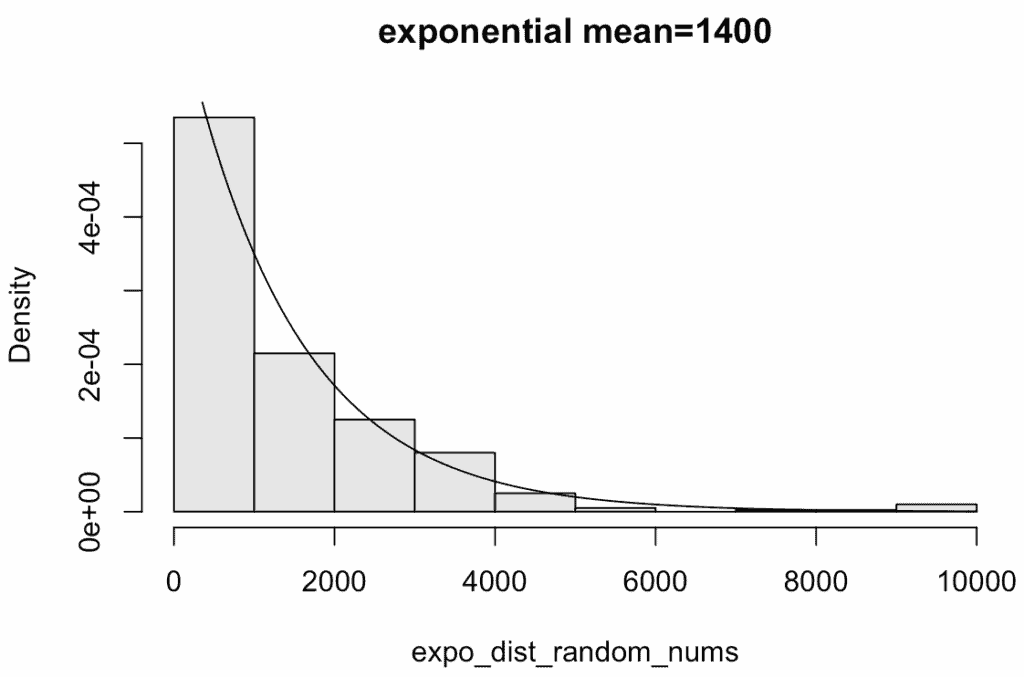# How to Generate Exponentially Distributed Random Numbers in R

The exponential distribution is a continuous probability distribution that defines the time between events in a process that occurs continuously and independently at a constant average rate.

To generate exponentially distributed random numbers, use the “rexp()” function. It returns the values from the exponential distribution.

It accepts the following two parameters.

1. observations: It takes observations you want to see.
2. rate: It is the estimated rate of events for the distribution, usually 1/expected service life or wait time.

## Visual representation## Example

``````set.seed(99)

expo_dist_random_nums <- rexp(10, rate = 0.6)

print(expo_dist_random_nums)``````

Output

``````  0.28237284 4.25622953 0.11664528 0.34030952 1.87766520 2.98135398
 0.16272462 0.01817234 3.22831023 0.45634704``````

In this example, we passed ten as observations and rate = 0.6; we got the output of 10 exponentially distributed random numbers.

## Generating a histogram based on an exponential distribution

To create a histogram, use the “hist()” function and pass the exponential random numbers, probability=TRUE, col = gray(.9), main=”exponential mean=1400″ arguments.

``````set.seed(99)

expo_dist_random_nums <- rexp(400, rate = 1/1400)

hist(expo_dist_random_nums, probability=TRUE, col= gray(.9),
main="exponential mean=1400")